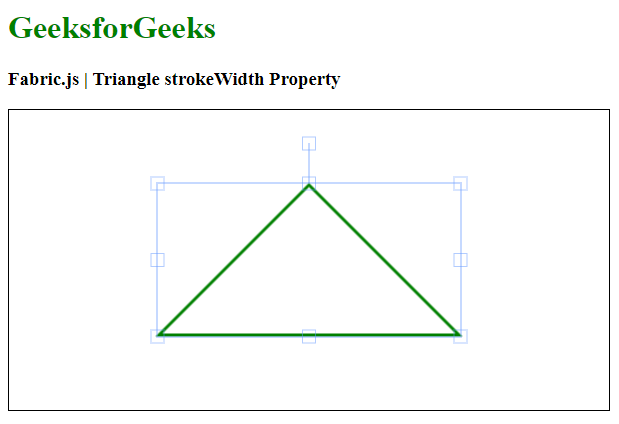Open in App
Not now

# Fabric.js Triangle strokeWidth Property

• Last Updated : 20 Jan, 2021

In this article, we are going to see how to set the stroke width of canvas Triangle using FabricJS. The canvas means Triangle is movable and can be stretched according to requirement. Further, the Triangle can be customized when it comes to initial stroke color, fill color, stroke width, or radius.

Approach:

• To make it possible we are going to use a JavaScript library called FabricJS.
• After importing the library using CDN, we will create a canvas block in the body tag which will contain our Triangle.
• After this, we will initialize instances of Canvas and Triangle provided by FabricJS and set stroke width of a canvas Triangle using strokeWidth property and render the Triangleon the Canvas as given in the example below.

Syntax:

```fabric.Triangle({
width: number,
height: number,
stroke: string
strokeWidth: number
});```

Parameters: This function accepts three parameters as mentioned above and described below:

• width: It specifies the width of triangle.
• height: It specifies the height of triangle.
• strokeWidth: It specifies the stroke width.

Example: This example uses FabricJS to set stroke strokeWidth of a canvas triangle.

## HTML

 ```<``html``>`` ` `<``head``>``    ``<``title``>``        ``Fabric.js | Triangle strokeWidth Property``    ```` ` `    ````    ``<``script` `src``=``"https://cdnjs.cloudflare.com/ajax/libs/fabric.js/3.6.2/fabric.min.js"``>``    `````` ` `<``body``>``    ``<``h1` `style``=``"color: green;"``>``        ``GeeksforGeeks``    ```` ` `    ``<``h3``>``        ``Fabric.js | Triangle strokeWidth Property``    ```` ` `    ``<``canvas` `id``=``"canvas"` `width``=``"600"` `height``=``"300"` `        ``style``=``"border:1px solid #000000"``>``    ```` ` `    ``<``script``>`` ` `        ``// Initiate a Canvas instance ``        ``var canvas = new fabric.Canvas("canvas");`` ` `        ``// Initiate a triangle instance ``        ``var triangle = new fabric.Triangle({``            ``width: 300,``            ``height: 150,``            ``fill: '',``            ``stroke: 'green',``            ``strokeWidth: 3``        ``});`` ` `        ``// Render the Triangle in canvas ``        ``canvas.add(triangle);``        ``canvas.centerObject(triangle);``    `````` ` ``

Output:My Personal Notes arrow_drop_up